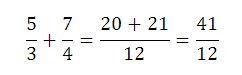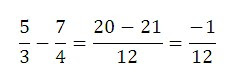# How to Add and Subtract Fractions Easily - Vedic Mathematics Methods

Hi friends, today we are going to learn one more simple and fast Vedic mathematics method for addition and subtraction of two fractions.

## Addition of two fraction numbers

In this method, we simply multiply crosswise and add them to get numerator of the the answer and multiply bottom (denominators) of two fractions to get the bottom of answer (see example).

Example 1:Multiply crosswise and add to get the numerator of the answer. Here 5×4=20 and 7×3=21. Then 20+21=41. The bottom of the fraction is just 3×4=12. So the answer is 41/12. Subtraction of two fraction numbers: Subtraction is same as that of addition; only the difference is that we have to subtract cross multiplication. In this method, we simply multiply crosswise and subtract them to get numerator of the answer and multiply bottom (denominators) of two fractions to get the bottom of the answer (see example).

## Subtraction of two fraction numbers

Example 1:Multiply crosswise and subtract to get the numerator of the answer. Here 5×4=20 and 7×3=21. Then 20-21=-1. The bottom of the fraction is just 3×4=12. So the answer is -1/12.

### You may also like

1.2.3.